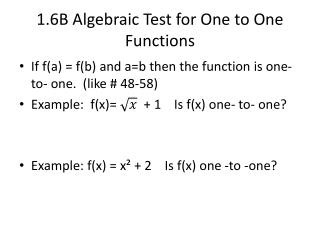Download Presentation1.6B Algebraic Test for One to O ne Functions1.6B Algebraic Test for One to O ne Functions - PowerPoint PPT Presentation

Download Presentation1.6B Algebraic Test for One to O ne Functions
An Image/Link below is provided (as is) to download presentation

Download Policy: Content on the Website is provided to you AS IS for your information and personal use and may not be sold / licensed / shared on other websites without getting consent from its author. While downloading, if for some reason you are not able to download a presentation, the publisher may have deleted the file from their server.

- - - - - - - - - - - - - - - - - - - - - - - - - - - E N D - - - - - - - - - - - - - - - - - - - - - - - - - - -
Presentation Transcript

1. 1.6B Algebraic Test for One to One Functions • If f(a) = f(b) and a=b then the function is one- to- one. (like # 48-58) • Example: f(x)= + 1 Is f(x) one- to- one? • Example: f(x) = x² + 2 Is f(x) one -to -one?

2. Draw inverse feature in Calculator • Put original in y= • 2nd, PRGM, 8:DrawInv, “VARS” → Y-Vars., 1:Function, choose original equation (Y₁ etc.), enter • 2nd, “mode”, 2nd, “PRGM”, 1:ClrDraw, enter,done

3. Examples • #59. f(x)=2x – 3 Find inverse algebraically. Use Calculator to graph f(x) and together. Describe the relationship.

4. More Examples • #69. f(x)= (x- 2)² Restrict the domain so f(x) is one- to- one and has a true inverse function. Find the inverse of f(x) algebraically. State domain & range of .

5. More examples • # 81-87 odd. • 81. (0) • 83. (f ○ g)(2) • 85. (g(0)) • 87. (g ○ )(2)